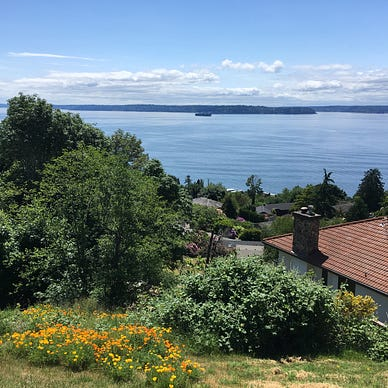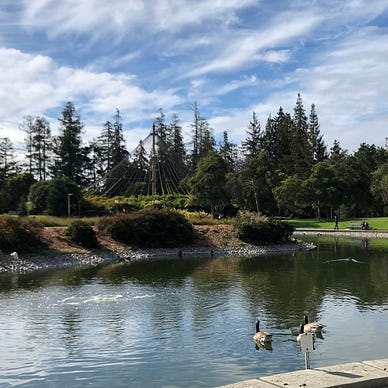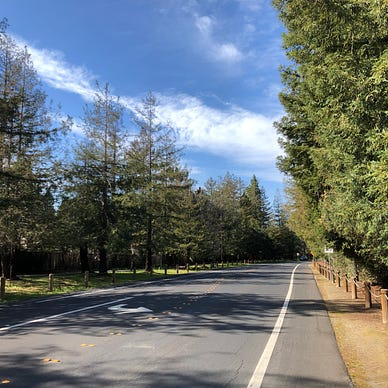# Plane Sweep for Roadmap Construction

Apply computational geometry to motion planning.

Plane sweep, as an algorithm paradigm, has found broad applications in computational geometric problems. In this post, I will introduce three applications of this powerful technique in robot motion planning.

# Line Segment Intersection

To begin with, let me introduce the plane sweep algorithm by looking at a classic problem. Given n line segments on a plane, find all their pairwise intersections assuming (1) no two segments are co-linear and (2) no three segments are intersected at the same point.

It would be not hard to give an O() brute force algorithm (enumerating every pair of segments) and claim that it has the best worst-case time complexity, since all pairs of the line segments may intersect. The advantage of a plane sweep algorithm is that it’s output-sensitive, meaning the runtime also depends on the output size and will be considerably lower than the worst case if output is sparse. Now let’s look at how it does so.

A key observation is that two line segments are possible to intersect only when their projections on the x-axis overlap, similar to the idea of the separating axis theorem (SAT). Without loss of generality, let’s assume no line segment is parallel to the y-axis. Now imagine there’s a vertical straight line, called the sweep line, scanning across the plane from x=-∞ to x=+∞. At each moment, we keep track of the line segments that are currently intersected with this sweep line, and thereby only consider intersections between these active line segments.

Although the movement of the sweep line is continuous, we can focus on just a finite number of interesting “events” — either an endpoint of a line segment we know a priori, or an intersection of two line segments that we catch on the fly. We need two balanced BSTs to maintain the information as we scan the plane: one for queuing the two types of events, and another for tracking the line sweep status — the line segments on the sweep line sorted by the y-coordinate.

In the beginning, we add all the endpoint events to the event queue. Then we iterate all the events while dynamically adding intersection events when we spot them. Every time we pop out an event, we update both the line sweep status and the event queue. Specifically, if it’s a left endpoint, we add the line segment to the status; if it’s a right endpoint, we remove the line segment from the status; if it’s an intersection of two line segments, we add it to the output set and exchange the order of the two segments in the sweep line status. After an event, if two line segments start neighboring, we add their pending intersection (if any) to the event queue; if two line segments no longer be neighbors, we remove their pending intersection (if any) from the event queue. The total number of events is 2*n+I, where I is the number of intersections. For each event, we need to do a constant number of O(log n)-time operations for status and event updates. Therefore, the total time complexity is O((n+I) log n).

As a coding exercise, one could try solving LC 1956 Minimum Time For K Virus Variants to Spread. At the time of writing this, a COVID-19 variant named Omicron is threatening the world.

# Configuration Space

Now let’s get into the realm of robot motion planning. If you never heard of the configuration space, this section is a gentle introduction

The configuration of a robot is a set of parameters that completely specify the position of every point in the robot system. For example, a 2D rigid body can be determined by three parameters: two for translation (x and y) and one for rotation (the yaw angle). A 3D rigid body needs six parameters: three for translation (x, y and z) and three for rotation (yaw, pitch and roll).

The configuration space of a robot is a (high-dimensional) manifold consisting of all the possible configurations, in contrast with the work space which is the physical world that the robot actually lives in. The configuration space of a 2D rigid body is a 3D manifold, and the configuration space of 3D rigid body is a 6D manifold.

In the configuration space, we don’t need to consider the complex joints and chains that exist in the work space. Instead, we can treat the robot as a single point in a high-dimensional space. The motion planning problem is essentially searching a path in this high-dimensional space that does not pass through any point that represents a configuration in collision with some obstacle in the work space. We call the set of all collision-free configurations as the free space.

Just as we would build a BGP-based backbone network to support the entire Internet, in motion planning we often build a roadmap in the free space for serving individual queries. A roadmap consists of a set of collision-free configurations and a set of collision-free connections between them. To serve a specific query, we just need to find two points A and B on the roadmap such that we know (1) how to travel from the start configuration to point A, (2) how to travel from point A to point B through the roadmap, and (3) how to travel from point B to the goal configuration. Common graph search algorithms, such as Dijkstra and A*, can help with (2).

The following sections will introduce three algorithms for constructing roadmaps in a 2D polygonal configuration space (i.e. obstacles in the configuration space are all polygonal).

# Vertical Cell Decomposition

Vertical cell decomposition is also known as trapezoidal decomposition. The idea is extending a line segment upwards and another downwards from each vertex of the configuration-space obstacles into the free space until hitting any other obstacle edges. By doing so, we partition the free space into trapezoids (or triangles for degenerate cases). Traveling from one vertical cell to its neighbor could be achieved by moving from the centroid of the first cell to the midpoint of their neighboring edge and then to the centroid of the second cell. In this way, we build the vertices and the edges of the roadmap.

Now let’s use the plane sweep algorithm to construct the decomposition, assuming all the vertices are in general positions (no two vertices have the same x-coordinate). As in the line segment intersection problem, we sweep a vertical line from left to right, stopping at each vertex (or event) in increasing order of their x-coordinates. Meanwhile, we maintain a balanced BST to track all the obstacle edges intersecting the sweep line. At each vertex, we could find the edges immediately above and below it (if any) by consulting the BST, so that we know where the two vertical extensions terminate. We also need to update the edges incident to the vertex in the BST. Specifically, we need to remove an edge if it’s to the left of the sweep line, and add an edge if it’s to the right of the sweep line.

In reality, plane sweep is seldom used for vertical cell decomposition in that it fails to help with another piece of puzzle required for serving planning queries — determining the cells containing the start configuration and the goal configuration respectively. That is a point location problem that can be addressed by randomized incremental construction¹, an alternative technique for building the trapezoidal map.

# Visibility Graph

One drawback to the vertical cell decomposition is that it cannot optimize the distance traveled from one cell to another. The visibility graph takes that into account. The observation is that the shortest path should be a polygonal path whose inner vertices can only be the vertices of the obstacles. Basically you don’t need to change your direction unless something blocks you. So in the visibility graph, we build the roadmap by connecting all the pairs of vertices that are visible to each other, meaning their straight line connection is not blocked by any obstacles.

The plane sweep algorithm once again comes into play when we try to find all the other vertices visible to a given vertex. Without this, a naive approach has to check each edge for every other vertex. Instead, we sweep a half-line emanating from the given vertex v by rotating it counterclockwise. Meanwhile, we keep track of the edges intersecting the sweep line in a balanced BST. The status of the intersecting edges only needs to be changed when we pass some vertex, since two edges can only be intersected at a vertex, in contrast with the general line segment intersection problem. As in the vertical cell decomposition, we stop at each vertex in the counterclockwise order, and decide if a vertex u is visible to v by checking whether there’s an edge in the balanced BST closer to u than v. When leaving a vertex, we also remove edges incident to it on the clockwise side of the sweep line, and add edges incident to it on the counterclockwise side of the sweep line.

In addition, there are a few corner cases that need attention. One is that we cannot add u as a vertex visible to v if their connection intersects the interior of the obstacle of which v is a vertex, even though there is no edge closer than u in the BST. Another is that there can be multiple vertices on the same sweep line. In that case, we need to process them in increasing order of distances to v, and regard farther vertices as invisible if a nearer vertex is already determined as invisible.

# Generalized Voronoi Diagram

The visibility graph gives us shortest paths in theory, but it’s impractical for real robots to bypass obstacles by nearly zero margin. A safe plan would keep enough clearance from all surrounding obstacles, which can be achieved by the approach introduced in this section.

A generalized Voronoi digram (GVD) is a set of points keeping equal distances from all surrounding obstacles (sites). In a polygonal free space, the GVD may consist of both line segments (bisectors between two edges) and parabolic arcs (points equidistant from an endpoint and an edge).

Using plane sweep to construct a GVD is quite convoluted, so I’ll just talk about an algorithm for a special case. The idea can be extended for the general case, though. In this special case, all the sites are mere points rather than polygons, and the structure made from this is called a (non-generalized) Voronoi digram, which consists of polygonal Voronoi cells. A Voronoi edge is a bisector between two sites, and a Voronoi vertex is the center of a circle passing through three or more sites.

This algorithm is known as Fortune’s algorithm. It sweeps a vertical line from left to right, as in line segment intersection, and it also keeps the sweep line status in a balanced BST. The difference, however, is that the sweep line status in this case is not about objects right on the sweep line, but about a beach line following the sweep line and constantly changing in shape. The beach line is defined as the set of parabolic curves that are equidistant from the sweep line and the rightmost sites to the left of the sweep line. At each moment, the portion of the diagram that is to the left of the beach line is completed, since no sites on the right side of the sweep line can be closer to them. But the portion between the beach line and the sweep line is still under construction. As the beach line moves forward, incomplete Voronoi edges stretch out until they meet together at new Voronoi vertices in pairs.

The sweep line status keeps track of the frontier sites that define the beach line sorted by the y-coordinate. Another balanced BST is used to store and poll two types of events that the algorithm iterates overs: (1) site events, in which the sweep line passes over new sites, and (2) circle events, in which the sweep line passes over new Voronoi vertices. A parabolic arc emerges on a site event and starts growing “flat” as the sweep line continues to go. Meanwhile, an arc starts diminishing as the two bisectors on its both sides converge, and vanishes at a new Voronoi vertex on a circle event. Circle events are anticipated when three sites become adjacent, either because of a new site or because of a vanishing arc, so they can be added to the event queue on the fly.

The Voronoi diagram is typically used for serving nearest neighbor queries besides its application in roadmap construction. Its dual graph, the Delaunay triangulation¹, is another versatile structure in computational geometry.

In summary, I talked about the plane sweep algorithm and its three applications for roadmap construction in motion planning.

Thanks for reading this! I hope you enjoy it. If you do, please also check out my other posts.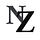## AI and Robotics

23 stories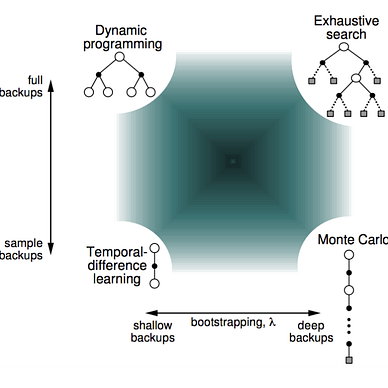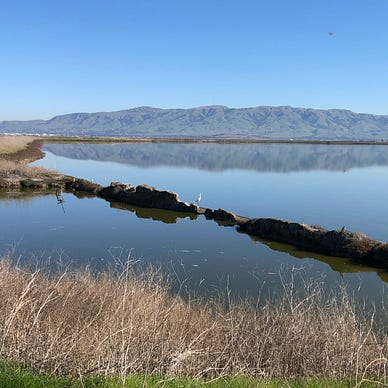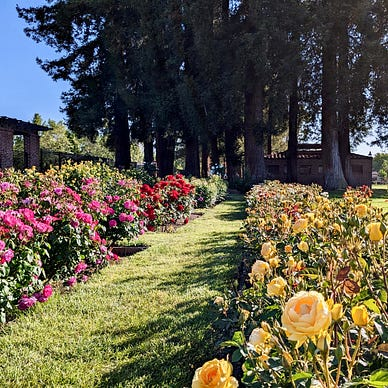## Cracking LeetCode Problems

7 stories